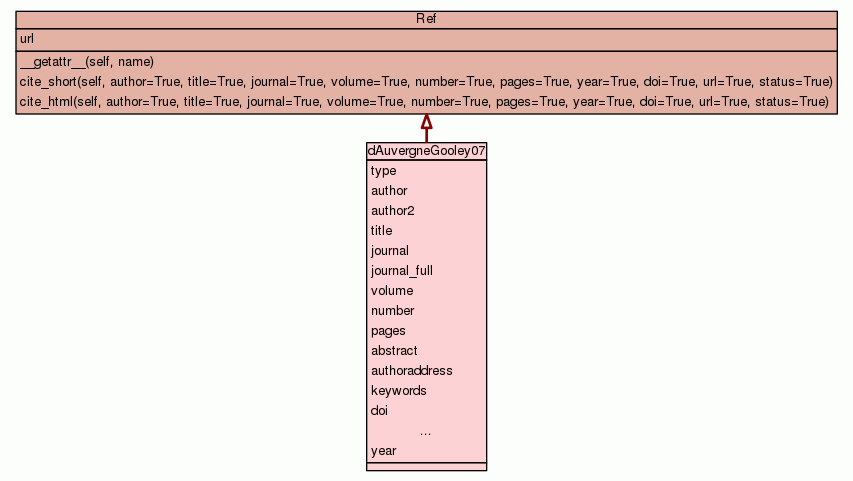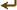[frames] | no frames]

# Class dAuvergneGooley07

source codeBibliography container.

 Instance Methods

Inherited from `Ref`: `__getattr__`, `cite_html`, `cite_short`

 Class Variables
type = `'journal'`
hash(x)
author = `'d\'Auvergne, E. J. and Gooley, P. R.'`
hash(x)
author2 = `[['Edward', 'd\'Auvergne', 'E.', 'J.'], ['Paul', 'Go...`
hash(x)
title = `'Set theory formulation of the model-free problem and ...`
hash(x)
journal = `'Mol. Biosys.'`
hash(x)
journal_full = `'Molecular BioSystems'`
hash(x)
volume = `'3'`
hash(x)
number = `'7'`
hash(x)
pages = `'483-494'`
hash(x)
abstract = `'Model-free analysis of NMR relaxation data, which ...`
authoraddress = `'Department of Biochemistry and Molecular Biol...`
keywords = `'Magnetic Resonance Spectroscopy/*methods ; *Models...`
doi = `'10.1039/b702202f'`
hash(x)
pubmed_id = `17579774`
hash(x)
status = `'published'`
hash(x)
year = `2007`
hash(x)

Inherited from `Ref`: `url`

 Class Variable Details

### author2

hash(x)

Value:
 ````[``[``'``Edward``'``, ``'``d\'Auvergne``'``, ``'``E.``'``, ``'``J.``'``]``,` `[``'``Paul``'``, ``'``Gooley``'``, ``'``P.``'``, ``'``R.``'``]``]` ```

### title

hash(x)

Value:
 ````'``Set theory formulation of the model-free problem and the diffusion se``eded model-free paradigm.``'` ```

### abstract

Value:
 ````'``Model-free analysis of NMR relaxation data, which describes the motio``n of individual atoms, is a problem intricately linked to the Brownian`` rotational diffusion of the macromolecule. The diffusion tensor param``eters strongly influence the optimisation of the various model-free mo``dels and the subsequent model selection between them. Finding the opti``mal model of the dynamics of the system among the numerous diffusion a``nd model-free models is hence quite complex. Using set theory, the ent``irety of this global problem has been encapsulated by the universal se``...` ```

 ````'``Department of Biochemistry and Molecular Biology, Bio21 Institute of ``Biotechnology and Molecular Science, University of Melbourne, Parkvill``e, Melbourne, Victoria 3010, Australia.``'` ```
 ````'``Magnetic Resonance Spectroscopy/*methods ; *Models, Theoretical ; Pro``teins/chemistry ; Thermodynamics``'` ```